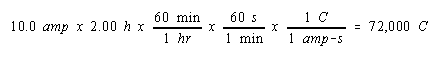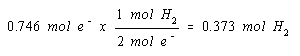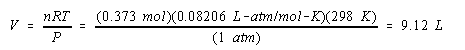Practice Problem 13

Calculate the volume of H2 gas at 25oC and 1.00 atm that will collect at the cathode when an aqueous solution of Na2SO4 is electrolyzed for 2.00 hours with a 10.0-amp current.

Solution

We start by calculating the amount of electrical charge that passes through the solution.We then calculate the number of moles of electrons that carry this charge.The balanced equation for the reaction that produces H2 gas at the cathode indicates that we get a mole of H2 gas for every two moles of electrons.

 Cathode (-): 2 H2O + 2 e-H2 + 2 OH-

We therefore get one mole of H2 gas at the cathode for every two moles of electrons that flow through the cell.We now have the information we need to calculate the volume of the gas produced in this reaction.